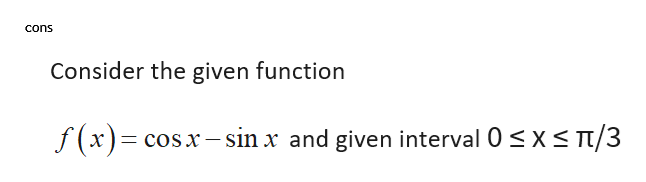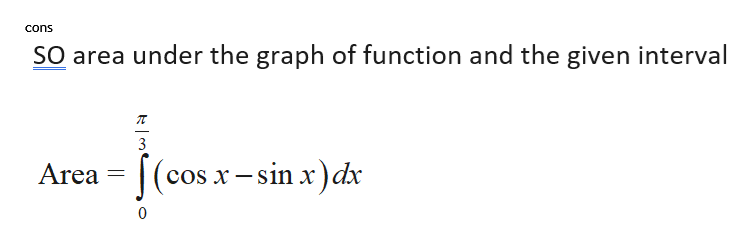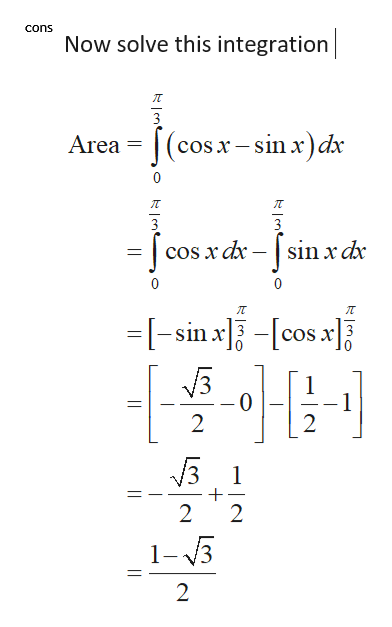# Let f (x) = cos x − sin x for 0 ≤ x ≤ π/3.Find the area A of the region between the graph of f and the x-axis on the given interval.

Question
27 views

Let f (x) = cos x − sin x for 0 ≤ x ≤ π/3.

Find the area A of the region between the graph of f and the x-axis on the given interval.

check_circle

Step 1help_outlineImage Transcriptionclosecons Consider the given function T/3 f(x)= cosx-sin x and given interval 0 s x fullscreen
Step 2help_outlineImage Transcriptionclosecons SO area under the graph of function and the given interval 3 (cos x-sin x)dx Area 0 fullscreen
Step 3help_outlineImage Transcriptionclosecons Now solve this integration 3 Area =(c cos x-sin x)dx 0 sin x dx 0 =-sin x-cos x 1 2 2 1 2 2 1-3 2 fullscreen

### Want to see the full answer?

See Solution

#### Want to see this answer and more?

Solutions are written by subject experts who are available 24/7. Questions are typically answered within 1 hour.*

See Solution
*Response times may vary by subject and question.
Tagged in

### Calculus